# 12.4 Discussion and Exercises

The running times of the depth-first-search and breadth-first-search algorithms are somewhat overstated by the Theorems 12.3 and 12.4. Define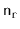as the number of vertices,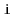, of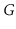, for which there exists a path fromto. Define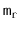as the number of edges that have these vertices as their sources. Then the following theorem is a more precise statement of the running times of the breadth-first-search and depth-first-search algorithms. (This more refined statement of the running time is useful in some of the applications of these algorithms outlined in the exercises.)

Theorem 12..5   When given as input a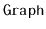,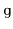, that is implemented using the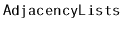data structure, the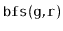,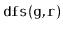and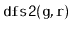algorithms each run in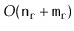time.

Breadth-first search seems to have been discovered independently by Moore  and Lee  in the contexts of maze exploration and circuit routing, respectively.

Adjacency-list representations of graphs were presented by Hopcroft and Tarjan  as an alternative to the (then more common) adjacency-matrix representation. This representation, as well as depth-first-search, played a major part in the celebrated Hopcroft-Tarjan planarity testing algorithm that can determine, in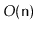time, if a graph can be drawn, in the plane, and in such a way that no pair of edges cross each other .

In the following exercises, an undirected graph is one in which, for everyand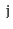, the edge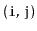is present if and only if the edge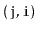is present.

Exercise 12..1   Draw an adjacency list representation and an adjacency matrix representation of the graph in Figure 12.7.Exercise 12..2   The incidence matrix representation of a graph,, is an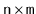matrix,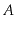, where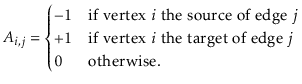1. Draw the incident matrix representation of the graph in Figure 12.7.
2. Design, analyze and implement an incidence matrix representation of a graph. Be sure to analyze the space, the cost of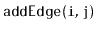,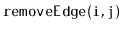,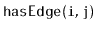,, and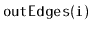.

Exercise 12..3   Illustrate an execution of the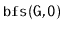and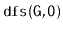on the graph,, in Figure 12.7.

Exercise 12..4   Letbe an undirected graph. We sayis connected if, for every pair of verticesandin, there is a path fromto(sinceis undirected, there is also a path fromto). Show how to test ifis connected in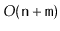time.

Exercise 12..5   Letbe an undirected graph. A connected-component labelling ofpartitions the vertices ofinto maximal sets, each of which forms a connected subgraph. Show how to compute a connected component labelling ofintime.

Exercise 12..6   Letbe an undirected graph. A spanning forest ofis a collection of trees, one per component, whose edges are edges ofand whose vertices contain all vertices of. Show how to compute a spanning forest of ofintime.

Exercise 12..7   We say that a graphis strongly-connected if, for every pair of verticesandin, there is a path fromto. Show how to test ifis strongly-connected intime.

Exercise 12..8   Given a graphand some special vertex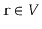, show how to compute the length of the shortest path from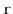tofor every vertex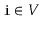.

Exercise 12..9   Give a (simple) example where thecode visits the nodes of a graph in an order that is different from that of thecode. Write a version ofthat always visits nodes in exactly the same order as. (Hint: Just start tracing the execution of each algorithm on some graph whereis the source of more than 1 edge.)

Exercise 12..10   A universal sink in a graphis a vertex that is the target of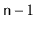edges and the source of no edges.12.1 Design and implement an algorithm that tests if a graph, represented as an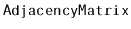, has a universal sink. Your algorithm should run intime.

#### Footnotes

... edges.12.1
A universal sink,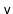, is also sometimes called a celebrity: Everyone in the room recognizes, butdoesn't recognize anyone else in the room.
opendatastructures.org